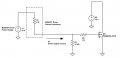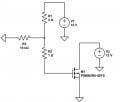# What is the maximum possible output current of the following equivalent circuit?

## What is the maximum possible output current of the following equivalent circuit?

• ### Output Current Calculation

• Total voters
0

#### vt600

Joined Jul 30, 2018
15
Following is the equivalent of the driver - MOSFET circuit.My intention was to prove the MOSFET-driver current driving capability. Driver is capable of drive up to 5 A. I am trying to calculate the maximum possible range of driver output current with following equivalent circuit.I am not quiet sure how can make an equivalent circuit model for the MOSFET in this case. Kindly suggest. I prefer to have a mathematical proof for the driver capability ( No issues with the theoretical conclusion, but more inclined to the mathematical proof)

This situation came in an evolving discussion about the MOSFET driver capability and the actual current required.

#### MrAl

Joined Jun 17, 2014
8,543
Hello,

By "maximum" do you mean the maximum that you SHOULD allow (due to mosfet limitations) or the maximum that can actually flow given you dont mind burning out the mosfet ?

•vt600

#### vt600

Joined Jul 30, 2018
15
Of course I can not let the MOSFET blown away. I am checking the maximum possible range of the current without damaging the MOSFET. And the intention to conclude mathematically that this current is under driver possible output current capacity (5 A)

#### MrChips

Joined Oct 2, 2009
24,593
If the power supply V2 has no current limit you will blow the MOSFET.
You need to either set a current limit on the power supply V2 or you have to provide a load resistor in series with the power supply.

•vt600

#### vt600

Joined Jul 30, 2018
15
This is an equivalent circuit of the actual case. In real case V2 is a transformer. Basically this is a part of the push pull converter. I am sorry for the confusion.
We can take any resistor (say 1 kΩ) for calculation purpose)
Could anyone suggest a method to find the driver maximum output current?

#### AnalogKid

Joined Aug 1, 2013
9,471
Are you asking about the peak current from the 10 V gate supply (charging the gate capacitance); or the steady-state current from the 12 V drain supply, presumably through some kind of load?

ak

#### MrChips

Joined Oct 2, 2009
24,593
Maximum output current = Vsupply / Rseries

where:

Rseries = Ron + Rload + Rsupply + Rwire

Ron = On resistance of MOSFET
Rsupply = internal resistance of supply
Rwire = resistance of interconnecting wires

#### vt600

Joined Jul 30, 2018
15
@ak, I am taking about the current from the 10 V gate supply

#### MrChips

Joined Oct 2, 2009
24,593
Why do you care about the gate supply?

The gate driver is being asked to deliver 10V / 10kΩ = 1mA.

#### ebp

Joined Feb 8, 2018
2,332

#### vt600

Joined Jul 30, 2018
15
Why do you care about the gate supply?

The gate driver is being asked to deliver 10V / 10kΩ = 1mA.
I believe you are ignoring the small resistance like 2 mΩ (Driver Internal resistance) and MOSFET internal resistance. I am looking for a solution considering even all the small resistances. I know this may seem bit disturbing but I would like to find the closest maximum output driver current. So I basically I was wondering how can approximate the MOSFET for finding the driver output current

#### vt600

Joined Jul 30, 2018
15

#### MrChips

Joined Oct 2, 2009
24,593
10V / 3Ω = 3.33A only if the MOSFET blows with the gate shorted to source pin.

•vt600

#### vt600

Joined Jul 30, 2018
15
Seems like if we can get an approximation for MOSFET circuit, can use kirchhoff's current law to find the voltage at the node at (R1, R2 and R3 comes). This will help to find the actual current at the driver output.

#### MrChips

Joined Oct 2, 2009
24,593
Seems like if we can get an approximation for MOSFET circuit, can use kirchhoff's current law to find the voltage at the node at (R1, R2 and R3 comes). This will help to find the actual current at the driver output.
Why bother?
Gate-Source resistance ≈ 10MΩ

Equivalent circuit resistance = 10kΩ

Gate driver current = 10V / 10kΩ = 1mA
as stated in post #9.

#### ebp

Joined Feb 8, 2018
2,332
I'm assuming we are talking about the maximum instantaneous current from the gate driver. The circuit clearly shows that the absolute maximum current will be 10/3 A. There is no mechanism to pull either source or drain below ground, so it can't be higher. It will in fact be lower by an amount that can only be known if the internal resistance of the FET's gate is known. That resistance is likely in the range of one or two ohms. If the internal resistance is represented by R2, then the abs max is 10/3 A. This is not steady state current, it is the peak current from the driver resulting from charging the gate capacitances. The peak current is influenced by the slew rate of the driver.

If you intend to quibble over miscellaneous milliohms, then show them in the schematic, otherwise I am entitled to conclude they don't exist.

The 10k resistor is just litter in terms of analyzing the circuit for peak current. The current through it is orders of magnitude too small relative to peak current to be worth considering. It is nearly the sole current path for steady-state current, in which case the other resistors are entirely irrelevant.

There are all sorts of good explanations of MOSFET input characteristics and behavior on the web from FET and driver manufacturers.

As drawn, the drain current is unlimited, so the FET will be destroyed very quickly.

#### Alec_t

Joined Sep 17, 2013
12,309
The gate-source part of a MOSFET behaves like a capacitor (typically > 1nF) in parallel with a resistor of many Megohms. That capacitor is discharged when the MOSFET is 'off' but has to be charged up to switch the MOSFET 'on'. Initially, Vgs = 0V, so the capacitor behaves effectively as a near short-circuit and the 10V supply has to provide an instantaneous current of 10V/(2Ω + 1Ω + a few milliohms) + 10V/10kΩ, i.e approximately 3.33A, as has been stated above.

#### crutschow

Joined Mar 14, 2008
28,153
As noted, the high current from the driver is only needed to rapidly charge and discharge the high gate capacitance of a MOSFET so that is switches rapidly and has low switching losses.
The MOSFET gate draws only insignificant DC leakage current, so the current for maintaining it in the ON state is negligible.